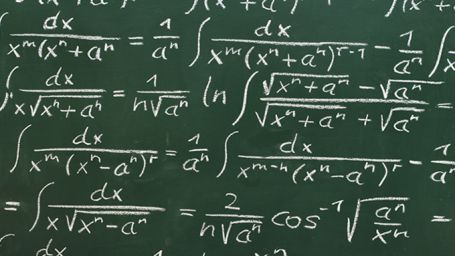Interactive

# Industrial Math: Measurements and Calculations

Martech
Updated Jan 20, 2021

After this lesson, you will be able to demonstrate how to use equations to solve industrial problems involving length, height, flow and temperature conversions and calculations. You will be able to explain how to read and interpret formulas, graphs, charts, and tables. You will also be able to apply several mathematical formulas to commonly used industrial calculations.

• Describe the use of conversion tables

• Use equations to solve problems working with length, height, and flow and temperature conversions

• Solve mathematical problems using each of the following:

– Area formulas

– Volumetric formulas

– Velocity formulas

– Flow formulas

– Density and specific gravity

• Explain how to interpret circle, bar, and line graphs

• Explain how to read and interpret charts and tables

;